Next: Model of Copernicus Up: Geometric Planetary Orbit Models Previous: Model of Hipparchus

# Model of Ptolemy

Ptolemy's geometric model of the motion of the center of an epicycle around a deferent can also be used to describe a heliocentric planetary orbit. The model is illustrated in Fig. 19. The orbit of the planet corresponds to the circle(only half of which is shown), where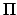is the perihelion point,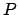the planet's instantaneous position, and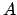the aphelion point. The diameter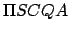is the effective major axis of the orbit, whereis the geometric center of circle,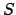the fixed position of the sun, andthe location of the so-called equant. The radius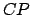of circleis the effective major radius,, of the orbit. The distances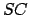and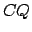are both equal to, whereis the orbit's effective eccentricity. The angle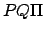is identified with the mean anomaly,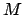, and increases linearly in time. In other words, as seen from, the planetmoves uniformly around circlein a counterclockwise direction. Finally,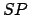is the radial distance,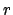, of the planet from the sun, and angle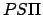is the planet's true anomaly,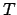.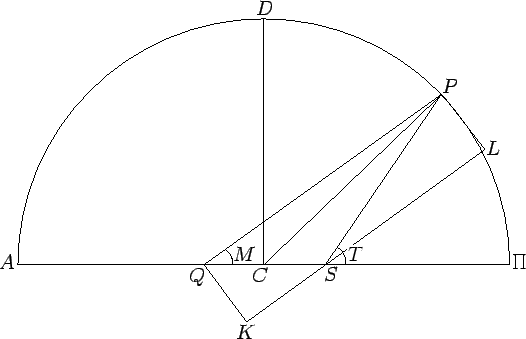Let us draw the straight-lineparallel to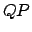, and passing through point, and then complete the rectangle. Simple geometry reveals that,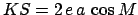, and, where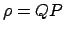. The cosine rule applied to triangle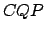yields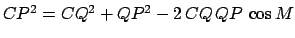, or, which can be solved to give. Moreover,, which implies that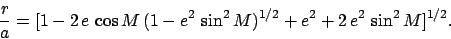(87)

Now,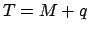, whereis angle. However,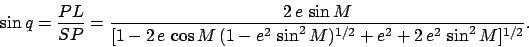(88)

Finally, expanding the previous two equations to second-order in the small parameter, we obtain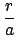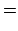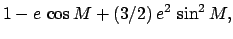(89)(90)

It can be seen, by comparison with Eqs. (81)-(82) and (85)-(86), that Ptolemy's geometric model of a heliocentric planetary orbit is significantly more accurate than Hipparchus' model, since the relative radial distance,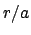, and the true anomaly,, in the former model both only deviate from those in the (correct) Keplerian model to second-order in.Next: Model of Copernicus Up: Geometric Planetary Orbit Models Previous: Model of Hipparchus
Richard Fitzpatrick 2010-07-21